Courses

# Facts That Matter - Pair of Linear Equations in TwoVariables Class 10 Notes | EduRev

## Class 10 : Facts That Matter - Pair of Linear Equations in TwoVariables Class 10 Notes | EduRev

The document Facts That Matter - Pair of Linear Equations in TwoVariables Class 10 Notes | EduRev is a part of the Class 10 Course Class 10 Mathematics by VP Classes.
All you need of Class 10 at this link: Class 10

Linear Equation in Two Variable

An equation that can be put in the form
ax + by + c = 0
where a, b and c are real numbers (a, b ≠ 0) is called a linear equation in two variables ‘x’ and ‘y’.

General Form of a Pair of Linear Equations in Two Variable

The general form of a linear pair of equations in two variables is:
a1x + b1y + c= 0  and
a2x + b2y + c2 = 0
where a1, b1, c1, a2, b2, c2 are real numbers such that the coefficients of x and y cannot be zero simultaneously.

Solution of a Pair of Linear Equations in Two Variables

The solution of a linear equation in two variables ‘x’ and ‘y’ is a pair of values (one for ‘x’ and other for ‘y’) which makes the two sides of the equation equal.
There are two methods to solve a pair of linear equations:
(i) Algebraic Method
(ii)Graphical Method.

Algebraic Method

There are 3 algebraic methods for solving a pair of linear equations in two variables.

(i) Substitution Method

Step 1: Find the value of one variable, say y in terms of the other variable, i.e., x from either equation, whichever is convenient

Step 2: Substitute this value of y in the other equation, and reduce it to an equation in one variable, i.e., in terms of x, which can be solved. Sometimes, you will get statements with no variables. If this statement is true, you can conclude that the pair of equations has infinitely many solutions. If the statement is false, then the pair of linear equations is inconsistent.

Step 3: Substitute the value of x (or y) obtained in Step 2 in the equation used in Step 1 to obtain the value of the other variable.

(ii) Elimination Method

Step 1: First, multiply both the equations by some suitable non-zero constants to make the coefficients of one variable (either x or y) numerically equal.

Step 2: Then add or subtract one equation from the other so that one variable gets eliminated. After you get an equation in one variable, go to step 3.

If in Step 2, you obtain a true statement involving no variable, then the original pair of equations has infinitely many solutions.

If in Step 2, you obtain a false statement involving no variable, then the original pair of equations has no solution, i.e., it is inconsistent.

Step 3: Solve the equation in one variable(x or y) so obtained to get its value.

Step 4: Substitute this value of x ( or y) in either of the original equations to get the value of the other variable.

(iii) Cross-Multiplication Method

Step 1: Write both of the given equations with their variables and constant term to the left-hand side.

Step 2: Write the coefficients of x,y and the constant term as: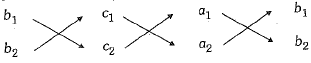Step 3: Now, obtain three equal fractions with numerators x, y and 1, for their denominators, multiply the numbers with downward arrows and from their product subtract the product of the numbers with upward arrows.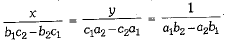Step 4: Now, obtain the value of x by equating the first and last term and the value of y by equating the second and last term. The values of x and y obtained above are the solution of the given equations.

Graphical Method

(i) If the graphs of two equations of a system intersect at a point, the system is said to have a unique solution, i.e., the system is consistent.
(ii) If the graphs of two equations of a system are two parallel lines, the system is said to have no solution, i.e., the system is inconsistent.
(iii) When the graphs of two equations of a system are two coincident lines, the system is said to have infinitely many solutions, i.e., the system is consistent and dependent.

Consistent and Inconsistent System

If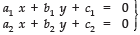form a pair of linear equations, then the following three situations can arise:
(i)   If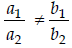, then the system is consistent.
(ii)  If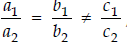, then the system is inconsistent.
(iii)  If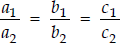, then the system is dependent and consistent.

Offer running on EduRev: Apply code STAYHOME200 to get INR 200 off on our premium plan EduRev Infinity!

132 docs

,

,

,

,

,

,

,

,

,

,

,

,

,

,

,

,

,

,

,

,

,

;